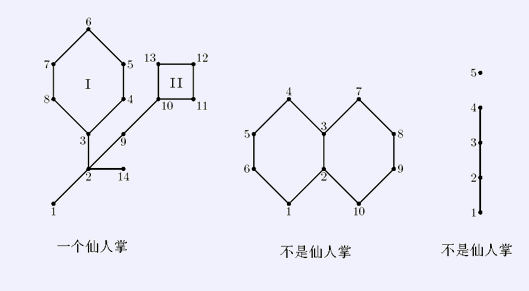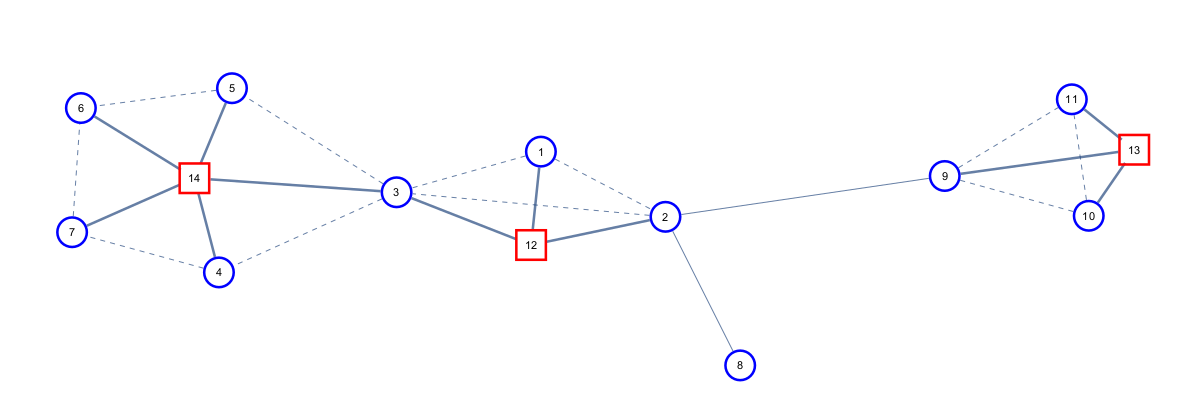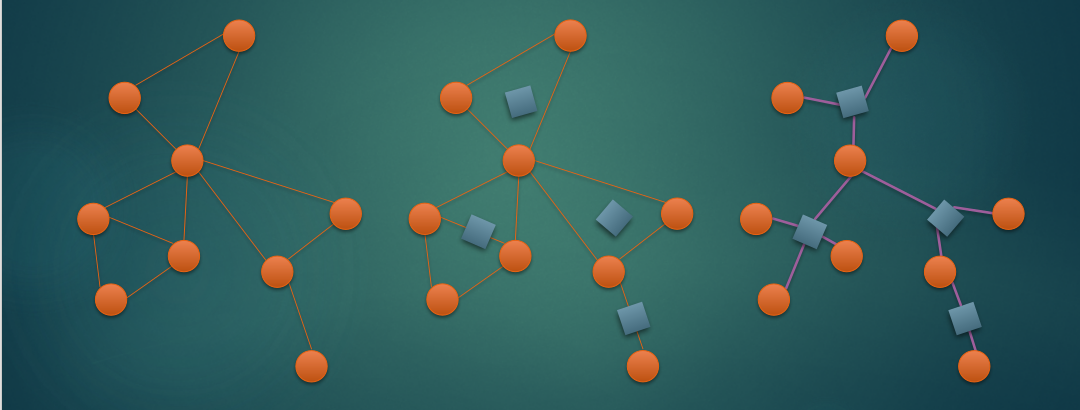# 圆方树浅谈

在联赛过后我又开始更博客啦。

### 一、前置知识点

首先在学习圆方树之前，要先有一些图论的基础，要先学会$tarjan$求点双。

### 二、仙人掌浅谈

首先先定义一下什么是仙人掌图。仙人掌图满足两个性质：性质一，仙人掌图是一个无向连通图。性质二，仙人掌图中的任意一条边最多存在于一个环中。借用$bzoj$的图来解释会清晰一点。我们考虑仙人掌图具有什么性质，将仙人掌图的$dfs$树取出，我们可以得到一课挂满非树边的树，这些非树边一定不相交。对于这个性质，我们可以解决一类问题，例如仙人掌的最大独立集问题。例题：bzoj4316: 小c的独立集

我们考虑上述的性质，即仙人掌中任意两条非树边不相交。因为上述性质我们可以知道，在求最大独立集的时候，任意两个环可以做到互不影响。所以我们可以先考虑不在环上的点，在将一个环上所有的点所引出的子树都考虑结束的时候，考虑整个环。我们设状态$f[i][0/1]$，表示对于$i$号点的子树中，第$i$号点选择/不选择的最大独立集大小是多少。现在我们考虑转移，对于一条树边，我们直接按照求树的最大独立集那样转移就可以。若不是树边，我们先不转移。由于每一个点是在处理完所有的子树内的点之后再被处理，所以我们发现，若现在要处理的是一个环的顶点（对于一个环的顶点的定义为这个环中最先遍历到的点），这个环上其他的所有点除了由当前环转移的部分，都已经处理完毕了，这个时候我们处理整个环。我们从整个环的最低端往上跳，我们只需要枚举当前环的最高点选择或不选择就好了，若环的最上方的点选择，整个环的最下方的点就不能选择，若环的最上方的点不选择，则环的最下方的点选择或不选择就是随意的。这样做的话，就相当于每一次将环单独拿出来考虑，其余的就变成树上的动态规划了。

这份代码咕掉啦


仙人掌的另一类题目，求直径，例题：bzoj1023：仙人掌图

我们考虑树的直径怎么求，我们还是将树边和环分开考虑，对于树边，我们直接像转移树的直径那样转移就可以了，对于环，我们考虑怎么更新$f[i]$（$f[i]$表示以$i$为端点，向$i$的子树中延伸的最长链）和答案，$f[i]$似乎很好考虑，$f[i]=Max\{f[j]+dis(i,j)]\}$。我们直接维护一下环上所有点的最大值就好了（对于$dis(i,j)$，我们只需要遍历一遍环就可以了）。考虑答案$ans=Max\{f[i]+f[j]+dis(i,j)\}$，对于上边的东西我们可以将$dis(i,j)$拆成$min(abs(dep[i]-dep[j]),cir_sze-abs(dep[i]-dep[j]))$，所以我们发现我们可以维护一个单调队列来更新答案。可能有人想问，这个不是单调栈就能维护的吗？我们考虑直径的定义，两点间的最短路径最长，所以我们要考虑在环上两个点之间要取最小距离，所以我们就要判断队首的点和当前的点的距离是否已经超过半个环的长度，若超过是要弹掉队首的。

#include <cstdio>
#include <algorithm>
using namespace std;
#define N 100010
int f[N],fa[N],ans=1,dep[N],low[N],cnt,level[N];
void dp(int p,int y)
{
int t=0;
for(int i=y;i!=p;i=fa[i]) Q1[++t]=i; Q1[++t]=p;
reverse(&Q1,&Q[t+1]);
for(int i=1;i<=t;i++) Q1[i+t]=Q1[i];
H=1,T=0;
for(int i=1;i<=t+t;i++)
{
while(H<=T&&i-Q[H]>t/2) ++H;
if(H<=T) ans=max(ans,f[Q1[i]]+f[Q1[Q[H]]]+i-Q[H]);
while(H<=T&&f[Q1[i]]-i>f[Q1[Q[T]]]-Q[T]) --T;
Q[++T]=i;
}
for(int i=y;i!=p;i=fa[i])
f[p]=max(f[p],f[i]+min(level[i]-level[p],1+level[y]-level[i]));
}
void dfs(int p,int from)
{
fa[p]=from,dep[p]=low[p]=++cnt,level[p]=level[from]+1;
{
if(!dep[to[i]])  dfs(to[i],p),low[p]=min(low[p],low[to[i]]);
else if(to[i]!=from) low[p]=min(low[p],dep[to[i]]);
if(low[to[i]]>dep[p]) ans=max(ans,f[p]+f[to[i]]+1),f[p]=max(f[p],f[to[i]]+1);
}
}
int main()
{
scanf("%d%d",&n,&m);
for(int i=1,k,a,b;i<=m;i++)
{
scanf("%d%d",&k,&a);
} dfs(1,0),printf("%d\n",ans);
}


### 三、圆方树浅谈

可能有人要问，这个题目不是圆方树浅谈吗？为什么要先讲仙人掌。因为圆方树可以求解一些仙人掌的题目。首先我们先引用一下$WC2017$的课件：

仙人掌$G=(V,E)$的圆方树$T=(VT,ET)$为满足以下条件的无向图：$VT=RT∪ST,RT=V,RT∩ST=∅$，我们称$RT$集合为圆点、$ST$集合为方点$∀e∈E$，若$e$不在任何简单环中,则$e∈ET$，对于每个仙人掌中的简单环$R$，存在方点$pR∈ST$，并且$∀p∈R$满足$(pR,p)∈ET$，即对每个环建方点连所有点.

看完上边的讲解，我再讲讲我对于圆方树的理解。对于一棵仙人掌的圆方树来说，上面一共有两种点，一类是圆点，另一类是方点。原仙人掌中所有的点都映射为圆方树中的圆点，圆方树中方点是我们后加进去的，表示为仙人掌中的一个环。对于圆方树的连边规则：对于原仙人掌中的一条边，若这条边不属于任何一个环中，这条边直接将原仙人掌中它的两个端点所映射的相连，若这条边属于环上，我们不会将这条边体现在圆方树中，我们把原仙人掌的一个环上的所有点都连向这个环所映射的方点。如图，我们将一个仙人掌转化为圆方树的效果图：知道了仙人掌的结构，怎么构建似乎就很好办了，我们首先用$tarjan$缩点，把每一个大小超过$1$的环的点都和一个方点相连，对于原来的树边，判断连接的两个点是不是在一个环中，若不是我们就连接在一起。

圆方树拥有几个小性质：

性质一：方点和方点不会直接相连，考虑定义，十分显然。

性质二：无论哪一个点为根，圆方树的形态不会改变，即圆方树是无根树。

性质三：以$r$为根的仙人掌上$p$的子仙人掌就是圆方树中以$r$为根时，$p$子树中的所有圆点。（子仙人掌：以$r$为根的仙人掌上的点$p$的子仙人掌是去除掉$p$到$r$的所有简单路径后，$p$所在的连通块）。

例题：bzoj2125：最短路

我们考虑先建出此仙人掌的圆方树，我们考虑对于圆方树上的边的边权，若当前边连接的是两个圆点，则当前边就是原仙人掌中的边权，若当前点连接的是圆点和方点，我们将其边权定义为圆点到当前环中深度最小的点的最短路径长度，这样我们维护两个点的最短距离就可以直接在圆方树上求$Lca$，然后求路径和就好了。但是这样的求法有一定的问题，我们考虑当$Lca$是圆点的时候这个求法没有问题，但是当$Lca$是方点的时候，我们就相当于都走到了方点所代表的环的最浅的点，但是并不一定就要都走到那里，所以我们要用倍增，求到$Lca$的下方的两个点，在计算答案的时候，就是路径和加上两点之间在环上的最短距离。具体看代码就好了：

#include <cstdio>
#include <vector>
#include <algorithm>
using namespace std;
#define N 100010
vector <int> have[N]; bool in[N],vis[N]; long long val2[N<<1],len[N],up_len[N],dis[N];
void dfs3(int p,int from)
{
++many;
for(int i=from;i!=p;i=fa2[i]) have[many].push_back(i);
have[many].push_back(p);
}
void tarjan(int p,int from)
{
fa2[p]=from,dep[p]=low[p]=++cnt,sta[++top]=p,vis[p]=in[p]=true;
{
if(!vis[to[i]]) tarjan(to[i],p),low[p]=min(low[p],low[to[i]]);
else low[p]=min(low[p],dep[to[i]]);
}
if(to[i]!=from&&fa2[to[i]]!=p&&dep[to[i]]>dep[p]) dfs3(p,to[i]);
}
void dfs(int p,int b)
{
}
void dfs_(int p,int b,int x,int from)
{
vis[p]=true;
{
if((!vis[to[i]])||(to[i]!=from&&to[i]==x)) len[b]+=val[i];
if(!vis[to[i]]) dfs_(to[i],b,x,p);
}
}
void dfs2(int p,int from)
{
fa[p]=from,level[p]=level[from]+1;
up_len[to2[i]]=val2[i],dfs2(to2[i],p);
}
long long find(int a,int b)
{
long long ans=0;
if(level[a]>level[b]) swap(a,b);
for(int i=19;~i;i--)
if(level[b]-level[a]>=(1<<i)) ans+=up_len[i][b],b=fa[i][b];
if(a==b) return ans;
for(int i=19;~i;i--)
if(fa[i][a]!=fa[i][b]) ans+=up_len[i][a]+up_len[i][b],a=fa[i][a],b=fa[i][b];
return fa[a]<=n?ans+up_len[a]+up_len[b]:
ans+min(abs(dis[a]-dis[b]),len[fa[a]]-abs(dis[a]-dis[b]));
}
int main()
{
scanf("%d%d%d",&n,&m,&q),tot=n;
tarjan(1,0);
for(int i=1,rt;i<=many;i++) if(have[i].size()>1)
{
++tot,rt=0;
for(int j=0;j<(int)have[i].size();j++)
vis[have[i][j]]=0,rt=(!rt)||(dep[have[i][j]]<dep[rt])?have[i][j]:rt;
dfs_(rt,tot,rt,0);
for(int j=0;j<(int)have[i].size();j++) vis[have[i][j]]=0;
dfs(rt,tot);
}
dfs2(1,0);
for(int i=1;i<=19;i++) for(int j=1;j<=tot;j++)
fa[i][j]=fa[i-1][fa[i-1][j]],up_len[i][j]=up_len[i-1][j]+up_len[i-1][fa[i-1][j]];
for(int i=1,a,b;i<=q;i++) scanf("%d%d",&a,&b),printf("%lld\n",find(a,b));
}


### 四、广义圆方树

圆方树不一定就只能求仙人掌上的问题，我们实际上可以将其扩展。一个无向连通图的圆方树上的圆点表示的是原图上的点，方点表示的是原图上的每一个点双，连边规则不变。但是考虑两个相邻的点也能构成一个点双，所以广义圆方树上不存在圆点和圆点连边的情况。找到一张图，辅助理解一下。建图似乎还是像仙人掌的那样，我们用$tarjan$求每一个点双，并连边。给出一道例题：Codeforces487E：Tourists

对于这道题目，我们先建出这个无向连通图的圆方树。我们考虑一个性质，就是一个点双中，不论从哪个点进入点双，从哪个点出点双，我们都一定能找到一条简单路径经过整个点双的最小点。对于这个性质，我们可以定义方点的点权就为他所代表的点双中所有的点的最小点权，因为方点代表的点双中的所有点所映射的圆点都会和方点连边，所以方点的权值就是他所连接的所有圆点的权值最小值，当然圆点的权值就是原来点的权值。我们考虑查询，实际上就是查询树上路径点权最小值，直接用树剖加线段树就能实现。考虑修改，对于我们上述定义的方点点权来看，我们每一次修改一个圆点的权值，就要跟着修改和当前圆点连接的所有方点的权值，时间复杂度显然可以卡为$O(n^2)$，所以我们考虑重新定义方点的权值。由于圆方树是一棵无根树，所以我们知道每一个点在固定根的情况下只会有一个父亲，这样我们将方点的定义更改为，方点的所有儿子的权值最小值。这样我们修改就能做到一个$log$的了，因为我们更改就只会更改当前圆点，和其父亲。对于查询，当路径上的方点不是$Lca$的时候，显然方点的父亲圆点也被计算到了路径当中，所以不影响答案，但是当方点为$Lca$的时候，我们就要在求完路径最小值的时候，将方点的父亲圆点算进去。

#include <set>
#include <cstdio>
#include <vector>
#include <algorithm>
using namespace std;
#define N 200010
#define inf 1000000000
vector <int> bel[N]; multiset <int> s[N]; multiset <int> ::iterator it;
int mn[N<<2],ord[N],level[N],fa[N],top[N],size[N],son[N],num[N],low[N],dep[N],sta[N],Top,tot,cnt; bool vis[N];
void tarjan(int p,int from)
{
low[p]=dep[p]=++cnt,vis[p]=true;
{
if(!vis[to[i]])
{
sta[++Top]=to[i],tarjan(to[i],p),low[p]=min(low[p],low[to[i]]);
if(low[to[i]]>=dep[p])
{
++tot;
while(sta[Top+1]!=to[i]) bel[sta[Top]].push_back(tot),Top--;
bel[p].push_back(tot);
}
} else low[p]=min(low[p],dep[to[i]]);
}
}
void dfs(int p,int from)
{
size[p]=1,fa[p]=from,level[p]=level[from]+1;
dfs(to2[i],p),size[p]+=size[to2[i]],son[p]=size[son[p]]<size[to2[i]]?to2[i]:son[p];
}
void dfs2(int p,int from)
{
top[p]=from,ord[p]=++cnt;
if(son[p]) dfs2(son[p],from);
}
void change(int p,int l,int r,int x,int y)
{
if(l==r) return mn[p]=y,void();
int mid=(l+r)>>1;
if(x<=mid) change(p<<1,l,mid,x,y);
else change(p<<1|1,mid+1,r,x,y);
mn[p]=min(mn[p<<1],mn[p<<1|1]);
}
int find(int p,int l,int r,int x,int y)
{
if(x<=l&&r<=y) return mn[p];
int mid=(l+r)>>1,tmp=inf;
if(x<=mid) tmp=min(tmp,find(p<<1,l,mid,x,y));
if(y>mid) tmp=min(tmp,find(p<<1|1,mid+1,r,x,y));
return tmp;
}
void init()
{
for(int i=1;i<=tot<<2;i++) mn[i]=inf;
if(level[i]<level[to2[j]]) s[i-n].insert(num[to2[j]]);
for(int i=1;i<=n;i++) change(1,1,tot,ord[i],num[i]);
for(int i=n+1;i<=tot;i++) change(1,1,tot,ord[i],*s[i-n].begin());
}
int main()
{
scanf("%d%d%d",&n,&m,&q),tot=n;
for(int i=1;i<=n;i++) scanf("%d",&num[i]);
tarjan(1,0),rebuild(),cnt=0,dfs(1,0),dfs2(1,1),init();
for(int i=1,a,b;i<=q;i++)
{
scanf("%s%d%d",kind,&a,&b);
if(kind=='C')
{
change(1,1,tot,ord[a],b);
if(!fa[a]) {num[a]=b;continue;}
it=s[fa[a]-n].lower_bound(num[a]),s[fa[a]-n].erase(it),num[a]=b;
s[fa[a]-n].insert(b),change(1,1,tot,ord[fa[a]],*s[fa[a]-n].begin());
}
else
{
ans=inf;
while(top[a]!=top[b])
{
if(level[top[a]]>level[top[b]]) swap(a,b);
ans=min(ans,find(1,1,tot,ord[top[b]],ord[b])),b=fa[top[b]];
}
if(level[a]>level[b]) swap(a,b);
ans=min(ans,find(1,1,tot,ord[a],ord[b]));
if(a>n) ans=min(ans,num[fa[a]]);
printf("%d\n",ans);
}
}
}
posted @ 2019-11-20 09:20  Yang1208  阅读(351)  评论(0编辑  收藏  举报